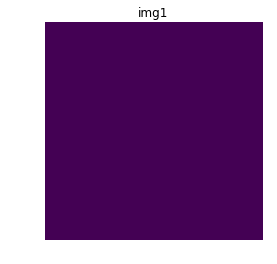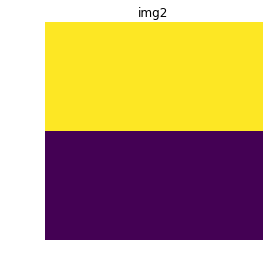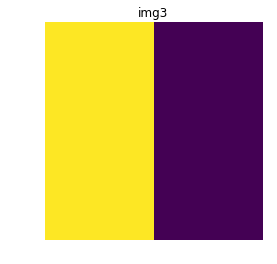# Introduction to Convolutions using Python

Convolutions are one of the key features behind Convolutional Neural Networks. For the details of working of CNNs, refer to Introduction to Convolution Neural Network.

Feature Learning
Feature Engineering or Feature Extraction is the process of extracting useful patterns from input data that will help the prediction model to understand better the real nature of the problem. A good feature learning will present patterns in a way that increase significantly the accuracy and performance of the applied machine learning algorithms in a way that would be impossible or too expensive by the machine learning itself. Feature learning algorithms find the common patterns that are important to distinguish between the wanted classes and extract them automatically. After this process, they are ready to be used in a classification or regression problem.
Let us consider a popular image classification problem, classification of images of a face and a non-face object. In the early days of computer vision, scientists tried to solve the problem by hand coding the detection algorithms of possible features of a human face like shape, eyes, nose, lips etc. This approach usually gave poor results because a face may appear in so many varieties, that it was not possible to account for even a significant fraction of the features. Just a simple change in lighting or orientation can bring about change in an image such that the algorithms were no longer able to detect faces.
In 1998, Yann Lecun introduced the concept of Convolutional Neural Networks which was capable of classifying images of handwritten characters with about 99% accuracy. The great advantage of Convolutional Neural Networks is that they are uncommonly good at finding features in images that grow after each level, resulting in high-level features in the end. The final layers (can be one or more) use all these generated features for classification or regression.
Convolution
Convolution is an operation that is performed on an image to extract features from it applying a smaller tensor called a kernel like a sliding window over the image. Depending on the values in the convolutional kernel, we can pick up specific patterns from the image. In the following example, we will demonstrate detection of horizontal and vertical edges in an image using appropriate kernels.

## Python3

 `import` `numpy as np ` `import` `matplotlib.pyplot as plt ` ` `  `# let img1 be an image with no features ` `img1 ``=` `np.array([np.array([``200``, ``200``]), np.array([``200``, ``200``])]) ` `img2 ``=` `np.array([np.array([``200``, ``200``]), np.array([``0``, ``0``])]) ` `img3 ``=` `np.array([np.array([``200``, ``0``]), np.array([``200``, ``0``])]) ` ` `  `kernel_horizontal ``=` `np.array([np.array([``2``, ``2``]), np.array([``-``2``, ``-``2``])]) ` `print``(kernel_horizontal, ``'is a kernel for detecting horizontal edges'``) ` ` `  `kernel_vertical ``=` `np.array([np.array([``2``, ``-``2``]), np.array([``2``, ``-``2``])]) ` `print``(kernel_vertical, ``'is a kernel for detecting vertical edges'``) ` ` `  `# We will apply the kernels on the images by ` `# elementwise multiplication followed by summation ` `def` `apply_kernel(img, kernel): ` `    ``return` `np.``sum``(np.multiply(img, kernel)) ` ` `  `# Visualizing img1 ` `plt.imshow(img1) ` `plt.axis(``'off'``) ` `plt.title(``'img1'``) ` `plt.show() ` ` `  `# Checking for horizontal and vertical features in image1 ` `print``(``'Horizontal edge confidence score:'``, apply_kernel(img1,  ` `                                            ``kernel_horizontal)) ` `print``(``'Vertical edge confidence score:'``, apply_kernel(img1,  ` `                                            ``kernel_vertical)) ` ` `  `# Visualizing img2 ` `plt.imshow(img2) ` `plt.axis(``'off'``) ` `plt.title(``'img2'``) ` `plt.show() ` ` `  `# Checking for horizontal and vertical features in image2 ` `print``(``'Horizontal edge confidence score:'``, apply_kernel(img2,  ` `                                            ``kernel_horizontal)) ` `print``(``'Vertical edge confidence score:'``, apply_kernel(img2,  ` `                                            ``kernel_vertical)) ` ` `  `# Visualizing img3 ` `plt.imshow(img3) ` `plt.axis(``'off'``) ` `plt.title(``'img3'``) ` `plt.show() ` ` `  `# Checking for horizontal and vertical features in image3 ` `print``(``'Horizontal edge confidence score:'``, apply_kernel(img3,  ` `                                            ``kernel_horizontal)) ` `print``(``'Vertical edge confidence score:'``, apply_kernel(img3,  ` `                                            ``kernel_vertical)) `

Output:

[ [ 2 2]
[-2 -2] ] is a kernel for detecting horizontal edges
[ [ 2 -2]
[ 2 -2] ] is a kernel for detecting vertical edgesHorizontal edge confidence score: 0
Vertical edge confidence score: 0Horizontal edge confidence score: 800
Vertical edge confidence score: 0Horizontal edge confidence score: 0
Vertical edge confidence score: 800

My Personal Notes arrow_drop_upCheck out this Author's contributed articles.

If you like GeeksforGeeks and would like to contribute, you can also write an article using contribute.geeksforgeeks.org or mail your article to contribute@geeksforgeeks.org. See your article appearing on the GeeksforGeeks main page and help other Geeks.

Please Improve this article if you find anything incorrect by clicking on the "Improve Article" button below.

Article Tags :

1

Please write to us at contribute@geeksforgeeks.org to report any issue with the above content.